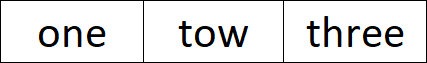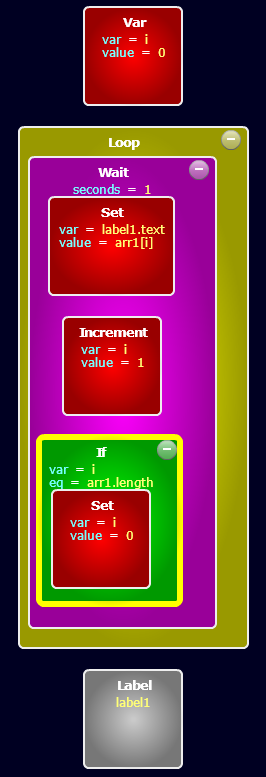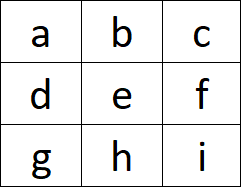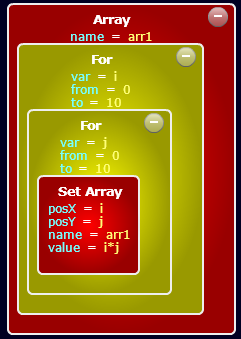#Cyberix3D Wiki

### Sidebar

editor:blocks:variables:array

# Array

An Array is a collection of values. You can use the Set Array command to store values in the array.
You can describe an Array in the following wayLet's say you want to create an Array with the values of “one”, “two” and “three”. You can do it in the following wayIn order to access the array values, you need to use the [] syntax.
arr1 value is “one”
arr1 value is “tow”
arr1 value is “three”

In order to know the Array length, you can use “.length” syntax.
arr1.length is 3

Here is an example of iterating through the arr1 array values and display them in a Labelhttp://www.gamemaker3d.com/player?pid=01615828

## 2D Array

A two-dimensional Array is a collection of Arrays. It means that every value in the array is an Array itself.
You can describe a 2D Array in the following wayYou can use the Set Array command to store values in the a 2D Array by using the x,y parameters as the cell position in the array.
You will need to set the following x,y position in order to build the above array
x=1, y=1 value = “a”
x=2, y=1 value = “b”
x=3, y=1 value = “c”
x=1, y=2 value = “d”
x=2, y=2 value = “e”
x=3, y=2 value = “f”
x=1, y=3 value = “g”
x=2, y=3 value = “h”
x=3, y=3 value = “i”

You can use the For command to build an array and set its values.
Here is an example of building a 2D Array with the multiplication tablehttp://www.gamemaker3d.com/player?pid=01615844

You can use the [][] syntax to access the 2D Array values
arr1 value is 0
arr1 value is 20
arr1 value is 15
.
.
.